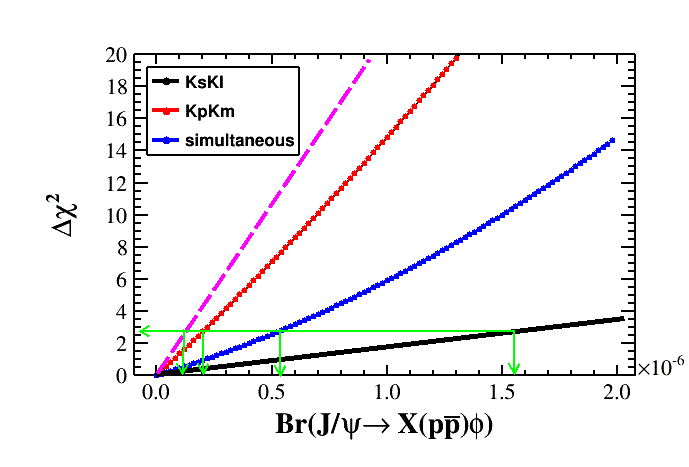# Combined upper limit of two data samples

Dear all,
I use the Least Square method in Roofit to do the upper limit on the branching fraction of two data samples: data1 (phi->KpKm) and data2 (phi->KsKl). The delta-chi2 v.s. the parameter Br is shown in the following plot:

for the fit of data1, the delta-chi2 is the red line; for the fit of data2, the delta-chi2 is the black line; for the simultaneous fit of data1 and data2, the delta-chi2 is the blue line. But, according to mathematical theory, chi2_sim = chi2_1 + chi2_2, and consider the minimum value, there is chi2_sim_min >= chi2_1_min + chi2_2_min . Therefore, for the simultaneous fit, the delta-chi2 should be higher than the red line, shown as the pink line. it could not be the blue line.

In other words, the combined upper limit should be less than the smaller one of the two data samples, right? (showing as the green arrows) who can hlep me ? thanks!Hi,

Yes, this looks strange to me. This could happen only when you have correlated data, so you cannot write the simultaneous likelihood as the sum of the two.
Maybe something wrong in the modelling? You could cross check this using RooStats

Best Regards

Lorenzo